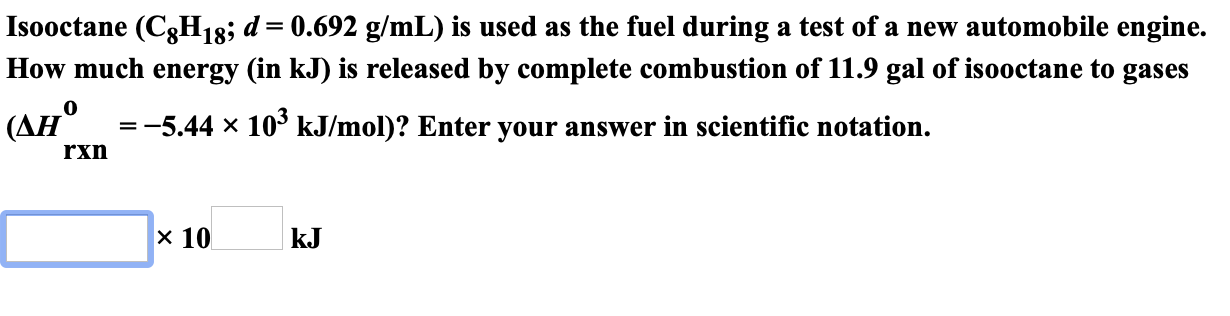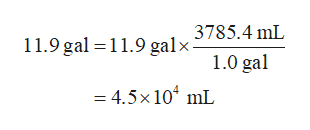Isooctane (C8H18; d= 0.692 g/mL) is used as the fuel during a test of a new automobile engine.How much energy (in kJ) is released by complete combustion of 11.9 gal of isooctane to gases(AH 5.44 x10° kJ/mol)? Enter your answer in scientific notation.rxnх 10kJ

Questionhelp_outlineImage TranscriptioncloseIsooctane (C8H18; d= 0.692 g/mL) is used as the fuel during a test of a new automobile engine. How much energy (in kJ) is released by complete combustion of 11.9 gal of isooctane to gases (AH 5.44 x 10° kJ/mol)? Enter your answer in scientific notation. rxn х 10 kJ fullscreen
Step 1

The energy released in the combustion of a hydrocarbon in a particular reaction is calculated by multiplying the number of moles of the hydrocarbon with the enthalpy change of the combustion reaction of the hydrocarbon.

The density of a substance is the ratio of its mass and volume. It is a measure of mass per unit volume. It is given by expression (1), in which, d is the density, m is the mass, and V is the volume of the substance.

The number of moles of a species is calculated by expression (4), in which, n is the number of moles, m is the mass, and M is the molar mass of the species.

Step 2

The conversion factor for gal and mL units is,

1 gal = 3785.4 mL

The volume of isooctane can be converted into mL units as,help_outlineImage Transcriptionclose11.9 gal 11.9 galx3785.4 mL 1.0 gal = 4.5x104 mL fullscreen
Step 3

The mass of isooctane in the given reactio...

Want to see the full answer?

See Solution

Want to see this answer and more?

Our solutions are written by experts, many with advanced degrees, and available 24/7

See Solution
Tagged in

Chemistry